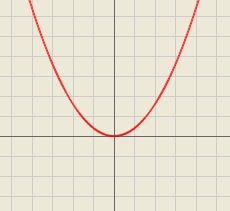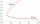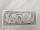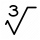# Evaluate 5

Evaluate expression x2−7x+12x−4 when x=−1

a =  -8

### Step-by-step explanation:Did you find an error or inaccuracy? Feel free to write us. Thank you!

Showing 1 comment:Matematik
for any expression we offer free fraction or decimal calculator that can solve (substitute variables and expression with variables evaluation):

https://www.hackmath.net/en/calculator/fraction?input=Evaluate+x2%E2%88%927x%2B12x%E2%88%924+when+x%3D%E2%88%921&submit=Calculate## Related math problems and questions:

• Eq2 2Solve following equation with quadratic members and rational function: (x2+1)/(x-4) + (x2-1)/(x+3) = 23
• Evaluate 17Evaluate 2x+6y when x=- 4/5 and y=1/3. Write your answer as a fraction or mixed number in simplest form.
• Simplify 3Simplify mixed numerals expression: 8 1/4- 3 2/5 - (2 1/3 - 1/4) Show your solution.
• If x=4If x=4 and y=-3, what is the value of the expression x² + 2xy - 4x + 3y + y²?
• Mixed numbersRewrite mixed numbers, so the fractions have the same denominator: 5 1/5 - 2 2/3
• Eq2 equationsFor each of the following problems, determine the roots of the equation. Given the roots, sketch the graph and explain how your sketch matches the roots given and the form of the equation: g(x)=36x2-12x+5 h(x)=x2-4x+20 f(x)=4x2-24x+45 p(x)=9x2-36x+40
• Turtles 2A box turtle hibernates in the sand at 11 5/8. A spotted turtle hibernates at 11 16/25 feet. Which turtle is deeper? Write answer as number 1 or 2.
• Sum of fractionsWhat is the sum of 2/3+3/5?
• Fraction + eqSolve following simple equation with fractions: -5/6(8+5b) = 75 + 5/3bThe heights of five starters on redwood high’s basketball team are 5’11”, 6’3”, 6’6”, 6’2” and 6’. The average of height of these players is?
• Equation 25Solve following simple equation: 3/4(x+5)=1/2(x+9)
• Profit, revenue, costProfit, P(x), is the difference between revenue, R(x), and cost, C(x), so P(x) = R(x) - C(x). Which expression represents P(x), if R(x) = 3x3 + 2x - 1 and C(x) = x4 - x2 + 2x + 3?
• Evaluate expr with 2 varsIf a=6 and x=2, find the value of (2ax + 7x- 10) /(4ax-3a-2)
• Evaluate expressionIf x=2, y=-5 and z=3 what is the value of x-2y
• BoatsThree-quarters of boats are white, 1/7 are blue and 9 are red. How many boats do we have?
• My fatherMy father has a big farm. 6/8 of it were planted with mango trees, 1/2 of the remainder are guava trees and the rest 10 trees are santol trees. What is the number of all trees?
• One third powerWhich equation justifies why ten to the one-third power equals the cube root of ten?